# What are non-canonical Hamilton systems

### 8.3 Canonical equation of motion - Hamilton's formulation

We define the Hamilton function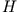of a mechanical system (use the definition of generalized momentum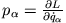)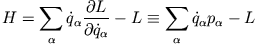and first want to show the conditions under which it is a conserved quantityUsing the Lagrange equation for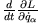and the chain rule for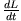One findsis therefore a conserved quantity if the Lagrange function does not explicitly depend on time. The Hamilton function is now the starting point for a reformulation of the equation of motion of classical mechanics. To do this, we consider the following partial derivatives ofThe resulting equations of motion for the generalized coordinates and momenta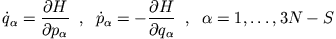one calls the Hamilton equations or the canonical equations of motion. They are equivalent to the Lagrange equations and thus also to Newton's equations of motion. The Hamilton function of a system of mass points is identical to the total mechanical energy if the constraints of the system are (i) holonomic, i.e. independent of the velocity of the mass points, and (ii) scleronomic, i.e. independent of time. In this case the generalized coordinates are only dependent on the Cartesian coordinates of the mass points, so that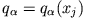: One can show that under such constraints the kinetic energy is a homogeneous quadratic function of the generalized velocity, i.e.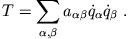So it follows

and you get for(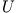is independent of the generalized speed)

Thereis a conservation quantity is also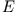a conserved quantity as we would expect from a conservative system.
M. Keim, H.J. Ludde